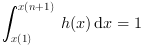Scilab Home page | Wiki | Bug tracker | Forge | Mailing list archives | ATOMS | File exchange
Change language to: English - Français - Português - 日本語 -

See the recommended documentation of this function

# histc

computes an histogram

### Calling Sequence

```[cf, ind] = histc(n, data [,normalization])
[cf, ind] = histc(x, data [,normalization])```

### Arguments

n

positive integer (number of classes)

x

increasing vector defining the classes (`x` may have at least 2 components)

data

vector (data to be analysed)

cf

vector representing the number of values of `data` falling in the classes defined by `n` or `x`

ind

vector or matrix of same size as `data`, representing the repective belonging of each element of data `data` to the classes defined by `n` or `x`

normalization

scalar boolean. `normalization=%f (default)`: `cf` represents the total number of points in each class, `normalization=%t`: `cf` represents the number of points in each class, relatively to the total number of points

### Description

This function computes a histogram of the `data` vector using the classes `x`. When the number `n` of classes is provided instead of `x`, the classes are chosen equally spaced and x(1) = min(data) < x(2) = x(1) + dx < ... < x(n+1) = max(data) with dx = (x(n+1)-x(1))/n.

The classes are defined by `C1 = [x(1), x(2)]` and `Ci = ( x(i), x(i+1)]` for i >= 2. Noting `Nmax` the total number of `data` (Nmax = length(data)) and `Ni` the number of `data` components falling in `Ci`, the value of the histogram for `x` in `Ci` is equal to Ni/(Nmax (x(i+1)-x(i))) when `"normalized"` is selected and else, simply equal to Ni. When normalization occurs the histogram verifies:when x(1)<=min(data) and max(data) <= x(n+1)

### Examples

• Example #1: variations around a histogram of a gaussian random sample
```// The gaussian random sample
d = rand(1, 10000, 'normal');

[cf, ind] = histc(20, d, normalization=%f)
// We use histplot to show a graphic representation
clf(); histplot(20, d, normalization=%f);

[cf, ind] = histc(20, d)
clf(); histplot(20, d);```• Example #2: histogram of a binomial (B(6,0.5)) random sample
```d = grand(1000,1,"bin", 6, 0.5);
c = linspace(-0.5,6.5,8);
clf()
subplot(2,1,1)
[cf, ind] = histc(c, d)
histplot(c, d, style=2);
xtitle("Normalized histogram")
subplot(2,1,2)
[cf, ind] = histc(c, d, normalization=%f)
histplot(c, d, normalization=%f, style=5);
xtitle("Non normalized histogram")```• Example #3: histogram of an exponential random sample
```lambda = 2;
X = grand(100000,1,"exp", 1/lambda);
Xmax = max(X);
[cf, ind] = histc(40, X)
clf()
histplot(40, X, style=2);
x = linspace(0, max(Xmax), 100)';
plot2d(x, lambda*exp(-lambda*x), strf="000", style=5)
legend(["exponential random sample histogram" "exact density curve"]);```• Example #4: the frequency polygon chart and the histogram of a gaussian random sample
```n = 10;
data = rand(1, 1000, "normal");
[cf, ind] = histc(n, data)
clf(); histplot(n, data, style=12, polygon=%t);
legend(["normalized histogram" "frequency polygon chart"]);```• histplot — plot a histogram
• hist3d — 3D representation of a histogram
• plot2d — 2D plot
• dsearch — поиск в упорядоченных наборах

### History

 Версия Описание 5.5.0 Introduction